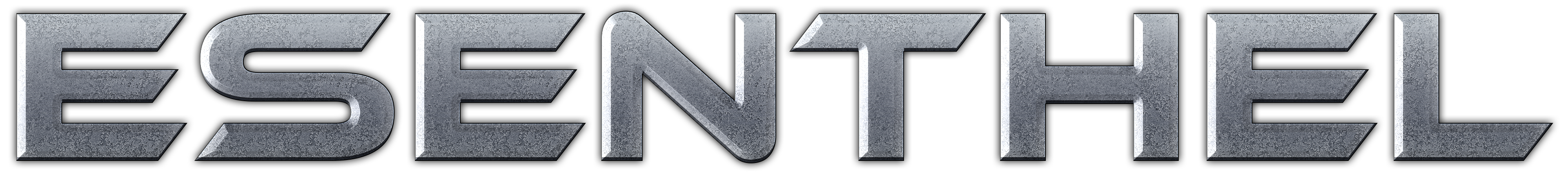About Store Forum Documentation ContactMovement and Camera Help. Author Message
PalaxeMember

 Post: #1 Movement and Camera Help. I have a question, I'm changing the movement in MMO. I have it set so left and right mouse button will move you. Right mouse button pans the camera and will steer you. Player Code: ```virtual Bool update()    {            if(action)       {          if(Ms.b(0) ||Kb.b(KB_A) || Kb.b(KB_D) || Kb.b(KB_Q) || Kb.b(KB_E))actionBreak();       }       if(!action)       {          // turn & move          input.turn.x=Kb.b(KB_Q)-Kb.b(KB_E);          input.turn.y=Kb.b(KB_T)-Kb.b(KB_G);          input.move.x=Kb.b(KB_D)-Kb.b(KB_A);          input.move.z=Ms.b(0) && Ms.b(1);                   input.move.y=Kb.b(KB_SPACE)-Kb.b(KB_LSHIFT);          // dodge, crouch, walk, jump          input.dodge = Kb.bd(KB_D)-Kb.bd(KB_A);          input.crouch= Kb.b (KB_LSHIFT);          input.walk  = Kb.b (KB_LCTRL );          input.jump  =(Kb.bp(KB_SPACE) ? 3.5 : 0);          // mouse turn          Flt max=DegToRad(900)*Time.d();          angle.x-=Mid(Ms.d().x*1.7, -max, max);          angle.y+=Mid(Ms.d().y*1.7, -max, max);              return super.update(); // call Game.Chr.update on which Player is based on``` So this seems to be working ok for what I'm after. The next part is where I'm having some difficulty. The camera is our side so your player will move and you see him turn. I want to put the camera so it stays focused on his head. I have tried all differn't ways but keep getting stuck. here is where I think it needs to be changed. Game Code: ```// update camera       if(Players.elms())       {          if(Ms.b(1))                   {                   Cam.yaw  -=Ms.d().x;             Clamp(Cam.pitch+=Ms.d().y, -PI_2, -0.1);          }          if(!Gui.ms() || Gui.ms()==Gui.desktop())          {             Clamp(Cam.dist*=ScaleFactor(Ms.wheel()*-0.2), 1, 20);          }          Cam.setSpherical(Players.ctrl.center(), Cam.yaw, Cam.pitch, 0, Cam.dist).updateVelocities().set();       }else       {          CamHandle(0.1, 300, CAMH_ZOOM|(Ms.b(1)?CAMH_MOVE:Ms.b(3)?CAMH_MOVE_XZ:Ms.b(4)?CAMH_ROT:0));       }``` I have tryed putting in Code: ```Vec head =plr.cskel.skeleton().getPoint(8"Head").pos+Vec(0, 0.18, -0.05); // calculate behind and above head position relative to body bone                if(plr.sac.body!=-1)head-=plr.cskel.skeleton().bones(plr.sac.body).pos;                head*=plr.cskel.scale();                Matrix3 look_matrix; look_matrix.setRotateXY(-plr.angle.y*0.6, -plr.angle.x); head*=look_matrix;                Vec pos=          plr.stored_pos+head;                    pos=Lerp(pos, plr.stored_pos             , 0.5f*plr.standCrouch());                    pos=Lerp(pos, plr.stored_pos+Vec(0,0.2,0),           CamTppCustom);                Cam.at=pos;``` I just keep running into so many errors. Is thier a better and easier way of doing this? Thanks for the help.
03-24-2013 06:35 AM
 « Next Oldest | Next Newest »